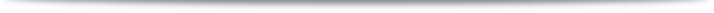﻿ Winter School on Higher Categories and TQFT
 Winter School on Higher Categories and TQFTTitles & Abstracts Home > Titles & Abstracts
Speaker : Gregory Ginot
Title : Higher Hochschild homology and factorization algebras.
Abstract : The goal of the lectures will be to explain how notions of
higher algebras can be applied to study or give examples of
topological or quantum field theories.
In the first part of the lecture we will define a generalization of
Hochschild (co)homology for commutative dg-algebras and explain its
relationship with mapping spaces and how to use it to produce a
2-dimensional topological field theory and higher dimensional
equivalent of genus 0 operation for them.
In the second part we will then explain a non-commutative version of
the above theory given by factorization algebras. We will describe
examples of this object coming from mathematical physics, using
sheaves of homotopy Lie algebras and derived critical locus.

Speaker :
Sangwook Lee
Title : Mirror symmetry of Calabi-Yau categories
Abstract : We recall (weak) Calabi-Yau category structures on Fukaya
categories and matrix factorization categories, which are mirror counterparts.
Then we investigate how we can compare these structures. This is based
on the joint work(in progress) with Cheol-hyun Cho and Hyung-Seok Shin.

Speaker : Junwu Tu
Title : homotopy L-infinity spaces and its applications
Abstract : L-infinity spaces were introduced by Kevin Costello in his study
of Quantum field theory. In this series of talks, I will tell a story of how
I arrived at this notion, and why it is useful in many different contexts.

References:
- E. Getzler, Lie theory for nilpotent L-infinity algebras. Annals of Mathematics, 170 (2009), 271301.
- K. Costello, A geometric construction of Witten genus, II, arXiv:1112.0816.
- J. Tu, Homotopy L-infinity spaces, arXiv:1411.5115, 2014.

Speaker : Gabriel Drummond-Cole
Title : Homotopy probability theory on a Riemannian manifold
Abstract : Homotopy probability theory is a homological enrichment
of algebraic probability theory, a toy model for the algebra of observables
in a quantum field theory. I will introduce the basics of the theory and
use it to describe a reformulation of fluid flow equations on a compact
Riemannian manifold.

Speaker :  Alexander Voronov
Title : Higher Categories and TQFTs
Abstract : A Topological Quantum Field Theory (TQFT) may be thought
of as a categorified cobordism invariant, providing a powerful tool for
studying manifolds and their cobordisms. In physics, one speaks about
state spaces and topologically invariant correlation functions.
All in all, TQFTs seem to stand at a high point of interaction between
mathematics and theoretical physics and are responsible for important
developments in both fields in the past thirty years. Higher categories and
Extended TQFTs came about as a generalization of open-closed 2d
Quantum Field Theory, sparkling many exciting ideas in topology
and mathematical physics. This minicourse will be an introduction
to TQFTs and higher categories, culminating in the cobordism hypothesis.

References:
- Mark Feshbach, Alexander A. Voronov, A higher category of cobordisms
and topological quantum field theory (arxiv:1108.3349
- Daniel S. Freed, The cobordism hypothesis. Bull. Amer. Math. Soc. 50 (2013),
no. 1, 57-92 (arXiv:1210.5100)
- Jacob Lurie, On the Classification of Topological Field Theories, Current Developments
in Mathematics, Volume 2008 (2009), 129-280 (arXiv:0905.0465)

Speaker : Jae-Suk Park
Title :  Quantization of the Rational Homotopy Theory
Abstract :  We explain how to associate a "classical field theory"  to
a topological space using a Sullivan algebraic model.
Finally, the possibility of establishing the concept of “fundamental group”
of quantum field theory will be discussed.

Speaker : Andrei Caldararu
Title : Algebraic structures on Hochschild invariants of algebraic varieties
and dg-categories
Abstract : Hochschild homology and cohomology are algebraic invariants
that can be defined in a wide variety of situations. They make sense for
algebraic varieties, orbifolds, Landau-Ginzburg models, and in general
whenever there is a natural dg-category associated to the corresponding
geometric object. In particular they can be thought of as one of a few “correct”
cohomology theories in non-commutative geometry. In my series of talks
I shall attempt to define them and discuss several ways to understand them.

a) Relationship to the deformation theory of associative algebras.
b) Description as differential forms/polyvector fields for a smooth
algebraic variety (the HKR isomorphism).
c) Interpretation as functions and distributions on the (algebraic)
free loop space of the underlying space. From this we will deduce
the existence of a circle action and briefly discuss the construction of
cyclic homology, as well as the E_2 algebra structure on cohomology.
d) Connections to Lie theory, in particular the Lie structure of TX[-1] given by
the Atiyah class, the Chern character, and applications of Kontsevich formality
to Riemann-Roch type theorems.

References:
- Weibel, “Introduction to Homological Algebra”, Cambridge University Press, Chapter 9.
- Amnon Yekutieli “The Continuous Hochschild Cochain Complex of a Scheme”,
arXiv:math/0111094
- N. Markarian, "The Atiyah class, Hochschild cohomology and the Riemann-Roch theorem,”
arXiv:math/0610553.
- M. Kapranov, "Rozansky-Witten invariants via Atiyah classes,” arXiv:alg-geom/9704009.
-  D. Calaque, M. Ven den Bergh, "Hochschild cohomology and Atiyah classes”,
arXiv:0708.2725